NEET  >  Projectile Motion

# Projectile Motion Notes | Study Physics Class 11 - NEET

## Document Description: Projectile Motion for NEET 2022 is part of Physics Class 11 preparation. The notes and questions for Projectile Motion have been prepared according to the NEET exam syllabus. Information about Projectile Motion covers topics like What is Projectile?, Frequently Asked Questions (FAQs) and Projectile Motion Example, for NEET 2022 Exam. Find important definitions, questions, notes, meanings, examples, exercises and tests below for Projectile Motion.

Introduction of Projectile Motion in English is available as part of our Physics Class 11 for NEET & Projectile Motion in Hindi for Physics Class 11 course. Download more important topics related with notes, lectures and mock test series for NEET Exam by signing up for free. NEET: Projectile Motion Notes | Study Physics Class 11 - NEET
 1 Crore+ students have signed up on EduRev. Have you?

What is Projectile?

• Projectile motion results from an object being thrown, shot, rolling off from a height, etc. and its in the air.  Once in the air, the only thing acting on the object is gravity.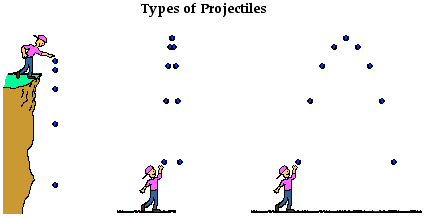• The path followed by a projectile is known as a trajectory. A ball thrown is an example of the projectile.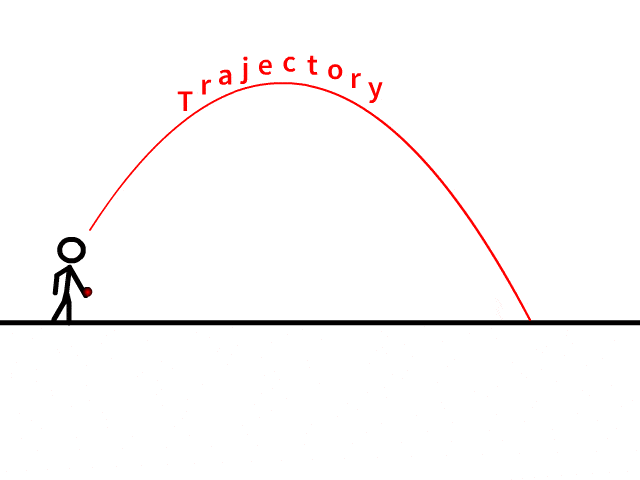What is Projectile Motion?

When a particle is thrown obliquely near the earth’s surface, it moves along a curved path under constant acceleration that is directed towards the centre of the earth (we assume that the particle remains close to the surface of the earth). The path of such a particle is called a projectile and the motion is called projectile motion.

In a Projectile Motion, there are two simultaneous independent rectilinear motions:

• Along the x-axis: uniform velocity, responsible for the horizontal (forward) motion of the particle.• Along y-axis: uniform acceleration, responsible for the vertical (downwards) motion of the particle.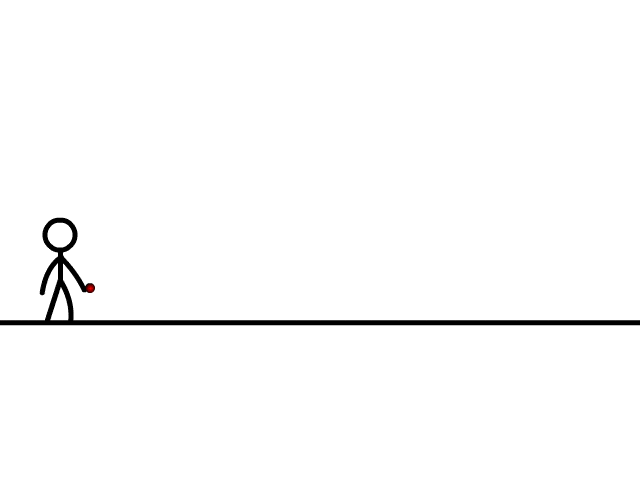• Acceleration in the horizontal projectile motion and vertical projectile motion of a particle: When a particle is projected in the air with some speed, the only force acting on it during its time in the air is the acceleration due to gravity (g). This acceleration acts vertically downward. There is no acceleration in the horizontal direction, which means that the velocity of the particle in the horizontal direction remains constant.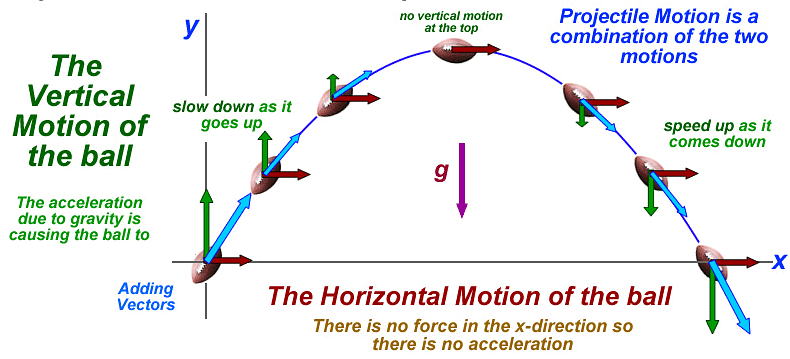Parabolic Motion of Projectiles

Let us consider a ball projected at an angle θ with respect to the horizontal x-axis with the initial velocity u as shown below: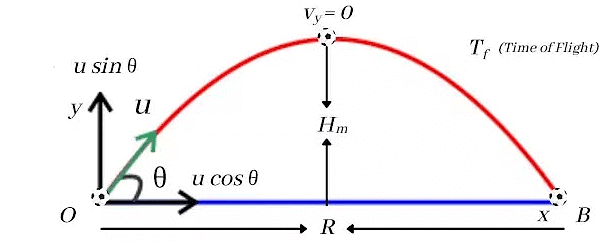• The point O is called the point of projection; θ is the angle of projection and OB = Horizontal Range or Simply Range.
• The total time taken by the particle from reaching O to B is called the time of flight.

For finding different parameters related to projectile motion, we can make use of differential equations of motions: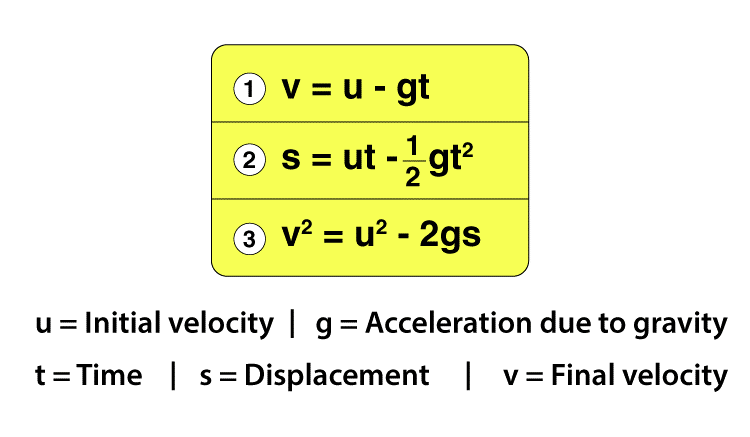(a) The Equation of Trajectory

Equation of Trajectory If x and y are the coordinates of particle after time t: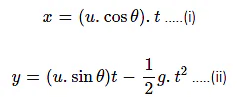From the equation (i) by putting the value of t, as a function of x, in equation (ii), we get: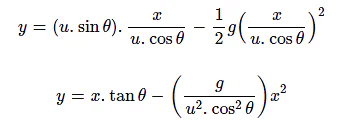The above equation is called the equation of trajectory. As the equation represents a parabola. Thus, the trajectory (or the path) of a projectile is a parabola

Tip: Here, u, θ, x and y are four variables. If any three quantities, as mentioned, are known then the fourth quantity can be solved directly

(b) Total Time of Flight

The time taken by a projectile to return its initial elevation after projection is known as time of flight.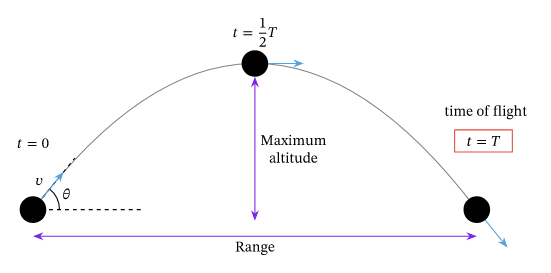It is denoted by (T) and given by: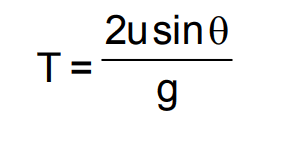(c) Maximum Height Attained

• The maximum vertical height traveled by the projectile during its journey is called the maximum height attained by the projectile.  It is the highest point of the trajectory.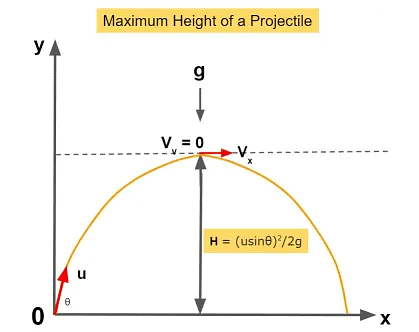• When the ball is at the highest point, the vertical component of the velocity will be zero. It is denoted by Hmax and given by: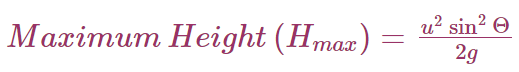Question for Projectile Motion
Try yourself:In a normal projectile motion, what will be the condition for maximum height?

(d) Horizontal Range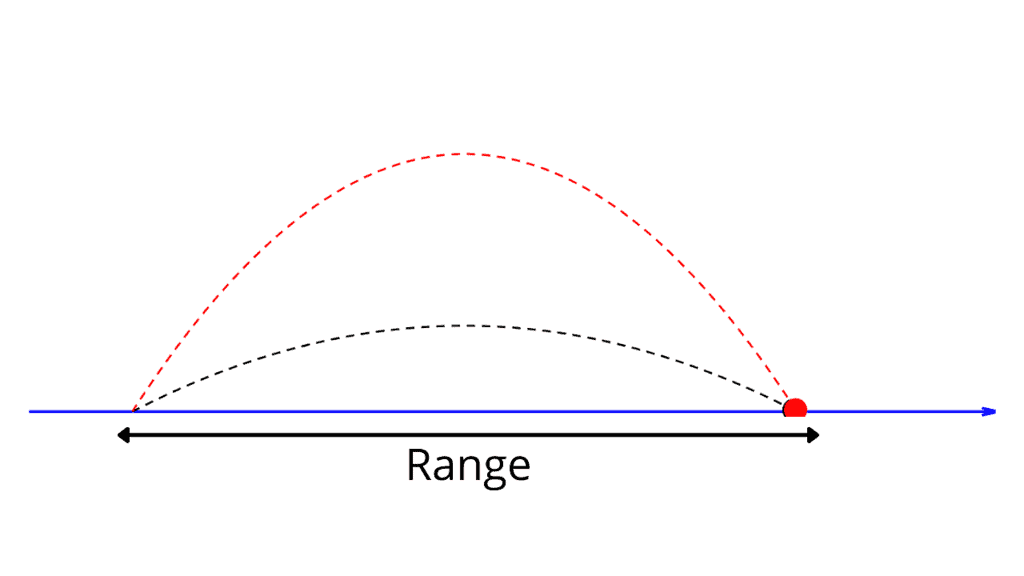Horizontal Range (OA) = Horizontal component of velocity (ux) X Total Flight Time (t)

R = u cos θ × (2usinθ)/g

Where,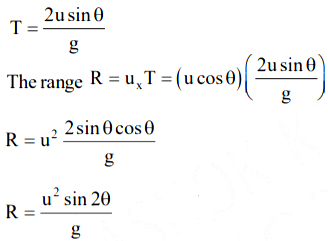Therefore, in a projectile motion the Horizontal Range is given by (R):Question for Projectile Motion
Try yourself:In a normal projectile motion, what will be the angle of the projectile to get the horizontal range minimum?

Question for Projectile Motion
Try yourself: In a normal projectile motion, what will be the condition for maximum range?

Solved Example

Q.1. An object of mass 2000 g covers a maximum vertical distance of 6 m when it is projected at an angle of 45° from the ground. Calculate the velocity with which it was thrown. Take g = 10 m/s2,

a) 12.10 m/s

b) 15.49 m/s

c) 2.155 m/s

d) 12.0 m/s

Ans: (a)

Solution

Here, h = 6

θ = 45°

g = 10

h = (v sinθ) 2/2g is the formula for maximum height.

Solving the equation by substituting the values,

h= (v2*[sin(45°)]2)/(2*10)

h = v2X (1/√2 )2/20

6 = [v2(1/2)]/20

6 X 20 X 2 = v2

240 = v2

v = 15.49

we get the initial velocity as 15.49 m/s.

Q.2. A body is projected with a velocity of 20ms−1 at 50° to the horizontal plane. Find the time of flight of the projectile.

Solution:

Initial Velocity Vo = 20ms−1

And angle θ=50°

So, Sin 50° =  0.766

And g= 9.8

Now formula for time of flight is:

T = 2⋅u⋅sinθ.g

T = 2×20×sin50°×9.8

= 2×20×0.766×9.8

= 30.64×9.8

T = 3.126 sec

Therefore time of flight is 3.126 second.

Frequently Asked Questions (FAQs)

Q.1. What is the concept of acceleration in horizontal and vertical projectile motion?

Ans: The only force acting on an object when it is projected in the air with some speed is the acceleration due to gravity (g). There is no acceleration in the horizontal direction because it acts vertically downwards, which means that the particle’s velocity remains constant in the horizontal direction.

Q.2. What is the trajectory?

Ans: A trajectory is known as the path followed by a projectile. When an object is thrown into space and upon which the only force acting is the force of gravity, then it is termed as a projectile. This doesn’t mean that other forces are not acting on it, but gravity is the primary force acting on a projectile. Due to this, the effects of other forces are minimised.

The document Projectile Motion Notes | Study Physics Class 11 - NEET is a part of the NEET Course Physics Class 11.
All you need of NEET at this link: NEET

## Physics Class 11

126 videos|449 docs|210 tests
 Use Code STAYHOME200 and get INR 200 additional OFF

## Physics Class 11

126 videos|449 docs|210 tests

### How to Prepare for NEET

Read our guide to prepare for NEET which is created by Toppers & the best Teachers

Track your progress, build streaks, highlight & save important lessons and more!

,

,

,

,

,

,

,

,

,

,

,

,

,

,

,

,

,

,

,

,

,

;